# A Photo Album with Python and PIL

January 4, 2010. Filed under python 59 pil 1

For a quick weekend adventure I decided to play around with using Python Imaging Library to take an arbitrary collection of photos and generate a photo gallery. With the help of the PIL documentation this turned out to be quite fun. (Full code available on Github.)

The pictures I'm editing are all my own, and come from my post about Kamioka, the town where I lived a couple years ago.

First, let's just load and resave an image without modifying it.

```>>> import Image
import Image
>>> img = Image.open("kamioka.png")
img = Image.open("kamioka.png")
>>> img.save("kam2.png")
img.save("kam2.png")
```

The saved image is identical to the original, which the kind reader likely hasn't yet seen.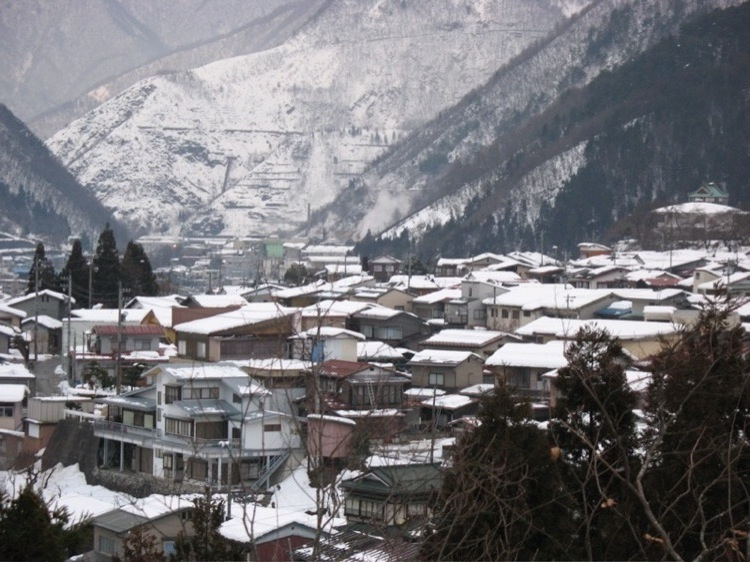I admit, not too exciting. Next, let's try creating a new image with two copies of that first image.

```def mirror(img, n=2):
x,y = img.size
mirror_img = Image.new("RGB", (x*n,y), "White")
for i in range(0, n):
mirror_img.paste(img, (i*x,0))
return mirror_img
```

Which we can then use to create an image with two copies.

```>>> img = Image.open("kamioka.png")
img = Image.open("kamioka.png")
>>> mirror(img).save("kam3.png")
mirror(img).save("kam3.png")
```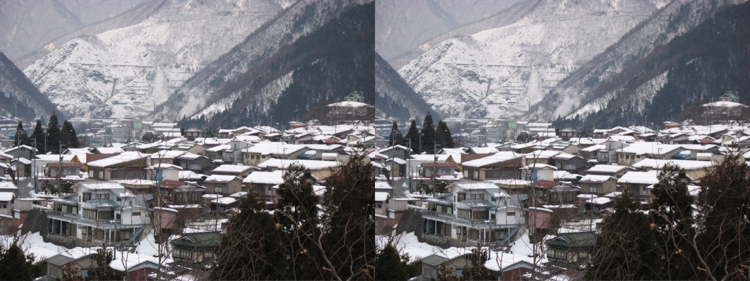We can also use it to make an image with a few more copies.

```>>> img = Image.open("kamioka.png")
img = Image.open("kamioka.png")
>>> mirror(img, n=5).save("kam3_2.png")
mirror(img).save("kam3_2.png")
```

Here it is with five copies.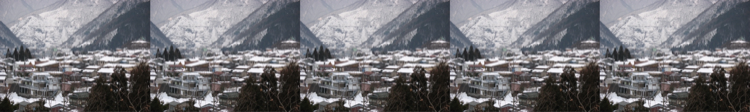Now, let's try creating borders for the pictures to add a bit of sophistication. Let's start with a monochrome border.

```def border(img, width=10, color="White"):
x,y = img.size
bordered = Image.new("RGB", (x+(2*width), y+(2*width)), color)
bordered.paste(img, (width, width))
return bordered
```

Here is a mirroring of a white bordered image.

```>>> img = Image.open("kamioka.png")
img = Image.open("kamioka.png")
>>> mirror(border(img)).save("kam4.png")
mirror(border(img)).save("kam4.png")
```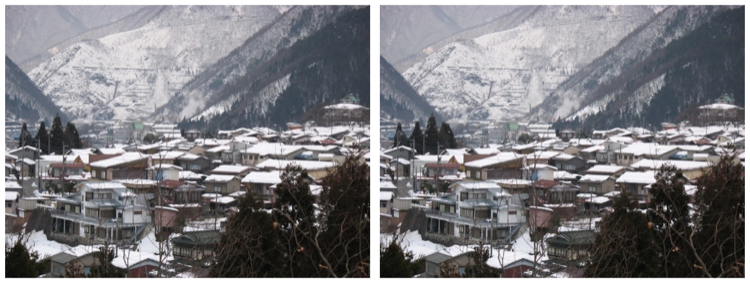I have to admit this is a pretty unimpressive border. The `border` function can be expanded to make it possible to customize borders a bit more. Instead of assuming a border has a width and a color, let's describe a border as a list of width/color tuples.

```def border(img, brdrs=[(2, "White"), (8, "Black"), (1, "Grey")]):
x,y = img.size
width = sum([ z for z in borders ])
bordered = Image.new("RGB", (x+(2*width), y+(2*width)), "Grey")
offset = 0
print offset
for b_width, b_color in brdrs:
bordered.paste(Image.new("RGB", (x+2*(width-offset),
y+2*(width-offset)), b_color), (offset, offset))
offset = offset + b_width
bordered.paste(img, (offset, offset))
return bordered
```

Once again calling `border`,

```>>> mirror(border(img)).save("kam5.png")
mirror(border(img)).save("kam5.png")
```

which brings us: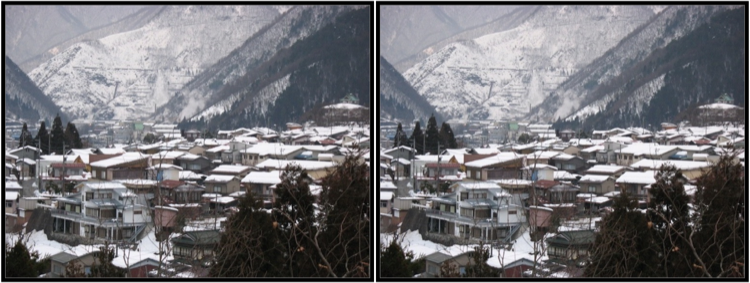Now that we've improved upon the border a bit, let's make a function which takes a list of images and displays them together neatly. To keep things simple, here are a few compromises we'll make:

• we'll scale all images to have the same height,
• we'll break pictures into chunks where the largest chunk is the size of the smallest original image,
• we'll tile the pictures uniformly.

First we need to normalize all the height of all images such that they are all the height of the shortest image.

```def normalize(imgs):
"Normalize height for all images to shortest image."
shortest = min([ x.size for x in imgs ])
resized = []
for img in imgs:
height_ratio = float(img.size) / shortest
new_width = img.size * height_ratio
img2 = img.resize((new_width, shortest), Image.ANTIALIAS)
resized.append(img2)
return resized
```

Next, we break wider images into chunks where each chunk is as wide as the skinniest image.

```def chunk(imgs):
"Break images into chunks equal to size of smallest image."
smallest = min([ x.size for x in imgs ])
height = imgs.size
chunked_imgs = []
for img in imgs:
parts = math.ceil((img.size * 1.0) / smallest)
for i in xrange(0, parts):
box = (i*smallest, 0, (i+1)*smallest, height)
img2 = img.crop(box)
chunked_imgs.append(img2)
return chunked_imgs
```

Third we need to tile the images into the album page. (Note that `merge` assumes images have been normalized and chunked.)

```def merge(imgs, per_row=4):
"Format equally sized images into rows and columns."
width = imgs.size
height = imgs.size
page_width = width * per_row
page_height = height * math.ceil((1.0*len(imgs)) / 4)
page = Image.new("RGB", (page_width, page_height), "White")
column = 0
row = 0
for img in imgs:
if column != 0 and column % per_row == 0:
row = row + 1
column = 0
pos = (width*column, height*row)
page.paste(img, pos)
column = column + 1
return page
```

Finally, we wrap up these function calls into the `album` function which uses them together and also adds a border.

```def album(imgs):
imgs = normalize(imgs)
imgs = chunk(imgs)
imgs = [ border(x) for x in imgs ]
return merge(imgs)
```

Now, creating the first album.

```>>> album(imgs).save("album.png")
album(imgs).save("album.png")
```

Here I used all the images from the Kamioka article, which happened to already be of the same sizes.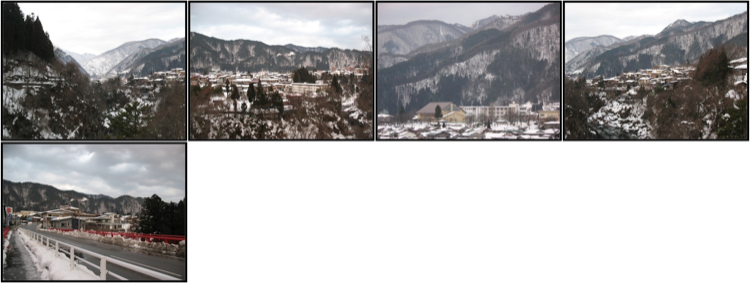And here is the output applied against the original images plus the output of the first call to `album`.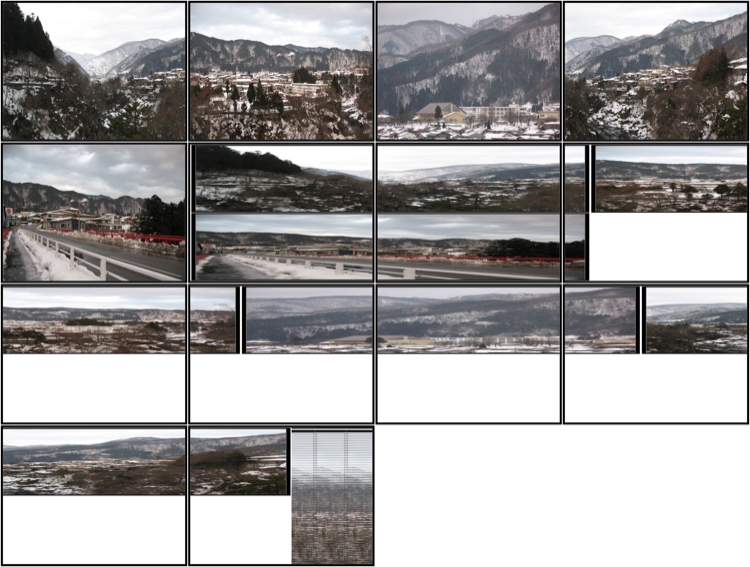So, that looks totally horrible, but with better choices of images it might actually look decent. I certainly enjoyed getting to work with PIL, and I hope some of the snippets from this meandering project serve as helpful examples.